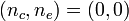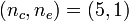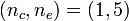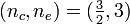Template:LSU HBook SSC Structure

Solution Strategies:

Detailed Force-Balance
(Introduction)

Virial Equilibrium
(Introduction)

 Example Solutions: Uniform-density sphere Isolated … ✓ ✓ Embedded in an External Medium … ✓ ✓ Polytropes Isolated … ✓ ✓ Embedded in an External Medium … ✓ ✓   🎦 Isothermal sphere Isolated … ✓ ✓ Embedded in an External Medium (Bonnor-Ebert Sphere) … ✓ ✓ Zero-temperature White Dwarf — Overview Power-law density distribution — Overview Other Analytically Definable Models ✓ … BiPolytropes (also referred to as Composite Polytropes) Overview Overview Core-Envelope Structure with$~(n_c,n_e) = (0,0)$ … ✓ ✓ Core-Envelope Structure with$~(n_c,n_e) = (5,1)$ … ✓ ✓ Core-Envelope Structure with$~(n_c,n_e) = (1,5)$ … ✓ … Core-Envelope Structure with$~(n_c,n_e) = (\tfrac{3}{2},3)$ … ✓ … Limiting Masses — Summary Instabilities Associated with Equilibrium Sequence Turning Points